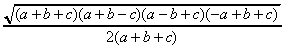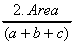# Pythagorean Triples

A Pythagorean triple is a set of three integers a, b, c which form the sides of a right angled triangle.

The smallest Pythagorean triple is the set of numbers 3,4,5.

What formula do a, b, c have to satisfy for the triangle to have a right angle opposite side C

This document requires an HTML5-compliant browser.
 BC AC AB Incircle Radius angle BAC

Here are some more Pythagorean triples: [6, 8, 10] [9, 12, 15] [12, 16, 20] [15, 20, 25] [18, 24, 30] [21, 28, 35]

Can you see how we created these? Can you create the next triple in the sequence?

Here is another sequence of Pythagorean triples:

[5, 12, 13] , [7, 24, 25] , [9, 40, 41] , [11, 60, 61] , [12, 35, 37] , [20, 21, 29], [20, 99, 101], [60, 91, 109], [15, 112, 113],
[47, 117, 125], [88, 105, 137], [17, 144, 145], [24, 143, 145]

Can you see how we created these? Can you create the next triple in the sequence?

Can you make more Pythagorean triples?

In Circle

Notice that when the triangle is defined by any of the triples above, the radius of the incircle is an integer.

Here is the formula for the radius of the incircle of a triangle whose sides are length a,b,c:This is closely related to Heron's formula for the area of a circle and can be rewritten like this:Verify that this formula gives an integer for the Pythagorean triples shown above.

Can you find any values for a, b, c which give an integer value for the incircle radius, but which are NOT a Pythagorean triple?

Can you prove that the incircle radius is an integer for all Pythagorean triples generated in the way shown above?

Can you prove that the incircle radius is an integer for all Pythagorean triples?

App generated by Geometry Expressions